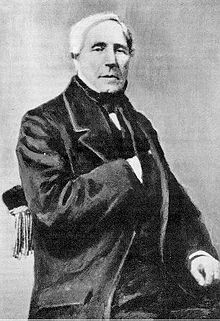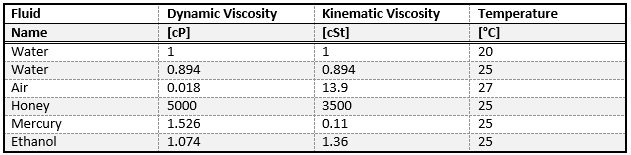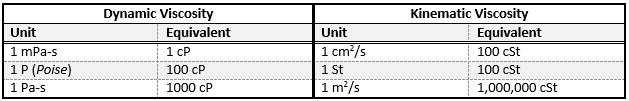# Viscosity Units

Common units for the two primary viscosity measurement types: dynamic and kinematic

### What Units Should I Use for Viscosity?

We get asked about the units of viscosity all the time. Sometimes it can be confusing since there are several types of viscosity, each with their own units. To further complicate things, different applications might use different unit systems such as SI, CGS....  In this page we briefly discuss the most common units for the two main types of viscosity: dynamic and kinematic.

### Units for Dynamic ViscosityThe most commonly used unit for dynamic viscosity is the CGS unit centipoise (cP), which is equivalent to 0.01 Poise (P). This unit is used in honor of French physicist, Jean Léonard Marie Poiseuille (1797-1869), who worked with Gotthilf Hagen on the widely known Hagen-Poiseuille law which applies to laminar flow through pipes. It is not a coincidence that the viscosity of distilled water at 20°C was used to define 1 cP! In order to give you an idea of the viscosity of some conventional fluids we have collected their viscosities in Table 1You can always check our application library to find examples of different fluids and their viscosity. The SI unit for dynamic viscosity η is the Pascal-second (Pa-s), which corresponds to the force (N) per unit area (m2) divided by the rate of shear (s-1). Just as in the definition of viscosity!However, since the viscosity of most fluids is below 1 Pa-s (See Table 1), the millipascal-second (mPa-s) is often used instead. Note that 1 mPa-s is equivalent to 1 cP.Table 1. Viscosity of Common Fluids

### Units for Kinematic ViscosityKinematic viscosity is often measured in the CGS unit centistokes (cSt), which is equivalent to 0.01 stokes (St). You guessed it! This one is named after Irish mathematician Sir George Gabriel Stokes (1819-1903) who, among other contributions to fluid mechanics, helped develop the Navier-Stokes equation for the conservation of momentum. One stoke is equivalent to one poise divided by the density of the fluid in g/cm3.The SI unit for kinematic viscosity is square meters per second (m2/s). However, due to the viscosity values of most common fluids, square centimeters per second (cm2/s) is used more often. Note that 1 cm2/s is equivalent to 100 cSt. In Table 2, we present most common units for viscosity and the conversion factors between them.Table 2. Conversion between common viscosity units.

These are the most basic units used for viscosity but there is a large variety of units that are specific to some particular measurement system or application. If you have further questions about what units to use for your viscosity measurements, contact us!

### Types of ViscosityWatch Webinar
Different Types of Viscosity